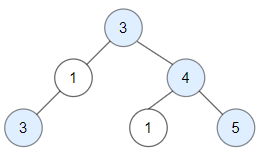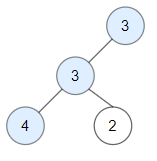# Count Good Nodes in Binary Tree

## 1: U-nderstand

Understand what the interviewer is asking for by using test cases and questions about the problem.

• Established a set (2-3) of test cases to verify their own solution later.
• Established a set (1-2) of edge cases to verify their solution handles complexities.
• Have fully understood the problem and have no clarifying questions.
• Have you verified any Time/Space Constraints for this problem?
• Can the input tree be Null?
• No, there will always be at least one node.
• Can a root-to-leaf path be the root itself, if it has no children?
• Yes, that can occur.
• What are the time and space constraints for this problem?
• `O(N)` time and `O(1)` space excluding the recusive stack.
``````HAPPY CASE
Input: root = [3,1,4,3,null,1,5]
Output: 4
Explanation: Nodes in blue are good.
Root Node (3) is always a good node.
Node 4 -> (3,4) is the maximum value in the path starting from the root.
Node 5 -> (3,4,5) is the maximum value in the path
Node 3 -> (3,1,3) is the maximum value in the path.````````````Input: root = [3,3,null,4,2]
Output: 3
Explanation: Node 2 -> (3, 3, 2) is not good, because "3" is higher than it.````````````EDGE CASE
Input: root = 
Output: 1
Explanation: Root is considered as good.``````

## 2: M-atch

Match what this problem looks like to known categories of problems, e.g. Linked List or Dynamic Programming, and strategies or patterns in those categories.

If you are dealing with Binary Trees some common techniques you can employ to help you solve the problem:

• Think about appropriate Tree Traversal: Pre-Order, In-Order, Post-Order, Level-Order
• Choosing a specific tree traversal that follows a general root-to-leaf path should help us identify all of the possible routes. However, we do need to process the node as we traverse down, so pre-order is helpful here.
• Store nodes within a HashMap to refer to later
• We don’t have a specific way of referring to previous nodes in a path that could be used in a HashMap. So, a HashMap would not help us as much in this context.
• Using Binary Search to find an element
• We are not working with a Binary Search Tree.
• Applying a level-order traversal with a queue
• Using this approach may complicate our code

## 3: P-lan

Plan the solution with appropriate visualizations and pseudocode.

General Idea: Lets collect the number of good nodes as we progress through the path by saving the largest value down the path and comparing the current node to the previous largest value.

``````1. Create a helper function to collect good nodes and retain the largest value down the path
a. Set basecase to root is None, return
b. Check current node value against previous max value
i. If a larger or equal value is found then increase good node count and set new max value
c. Recursively process left child and right child to progress through the tree
2. Create a variable to collect good nodes
3. Call helper function to populate good nodes
4. Return good nodes count``````

⚠️ Common Mistakes

• Choosing the wrong traversal type
• Try to walk through the problem by hand and see the order in which you are processing the nodes. This will clue you into the type of traversal necessary
• Not realizing the need for a helper function
• The helper function helps provide additional parameters and allow for retaining memory during recursion.

## 4: I-mplement

Implement the code to solve the algorithm.

``````# Definition for a binary tree node.
# class TreeNode:
#     def __init__(self, val=0, left=None, right=None):
#         self.val = val
#         self.left = left
#         self.right = right
class Solution:
def goodNodes(self, root: TreeNode) -> int:
# Create a helper function to collect good nodes and retain the largest value down the path
def findGoodNodes(root: TreeNode, maxValue: int):
nonlocal countGoodNodes

# Set basecase to root is None, return
if not root:
return

# Check current node value against previous max value
if root.val >= maxValue:
# If a larger or equal value is found then increase good node count and set new max value
countGoodNodes += 1
maxValue = root.val

# Recursively process left child and right child to progress through the tree
findGoodNodes(root.left, maxValue)
findGoodNodes(root.right, maxValue)

# Create a variable to collect good nodes
countGoodNodes = 0

# Call helper function to populate good nodes
findGoodNodes(root, root.val)

# Return good nodes count
return countGoodNodes``````
``````class Solution {
// Create a variable to collect good nodes
int count = 0;

public int goodNodes(TreeNode root) {
// Call helper function to populate good nodes
helper(root, root.val);
// Return good nodes count
return count;
}
// Create a helper function to collect good nodes and retain the largest value down the path
private void helper(TreeNode root, int max) {
// Set basecase to root is None, return
if (root != null) {
// Check current node value against previous max value
if (root.val >= max) {
// If a larger or equal value is found then increase good node count and set new max value
count++;
}
// Recursively process left child and right child to progress through the tree
helper(root.left, Math.max(root.val, max));
helper(root.right, Math.max(root.val, max));
}
}
}``````

## 5: R-eview

Review the code by running specific example(s) and recording values (watchlist) of your code's variables along the way.

• Trace through your code with an input to check for the expected output
• Catch possible edge cases and off-by-one errors

## 6: E-valuate

Evaluate the performance of your algorithm and state any strong/weak or future potential work.

Assume `N` represents the number nodes in tree

• Time Complexity: `O(N)` because we need to visit each node in binary tree.
• Space Complexity: `O(1)` if we do not count the recursion stack. The recursion stack will cost us `O(N)` if we have a linked list like tree. `O(logN)` in the average case.